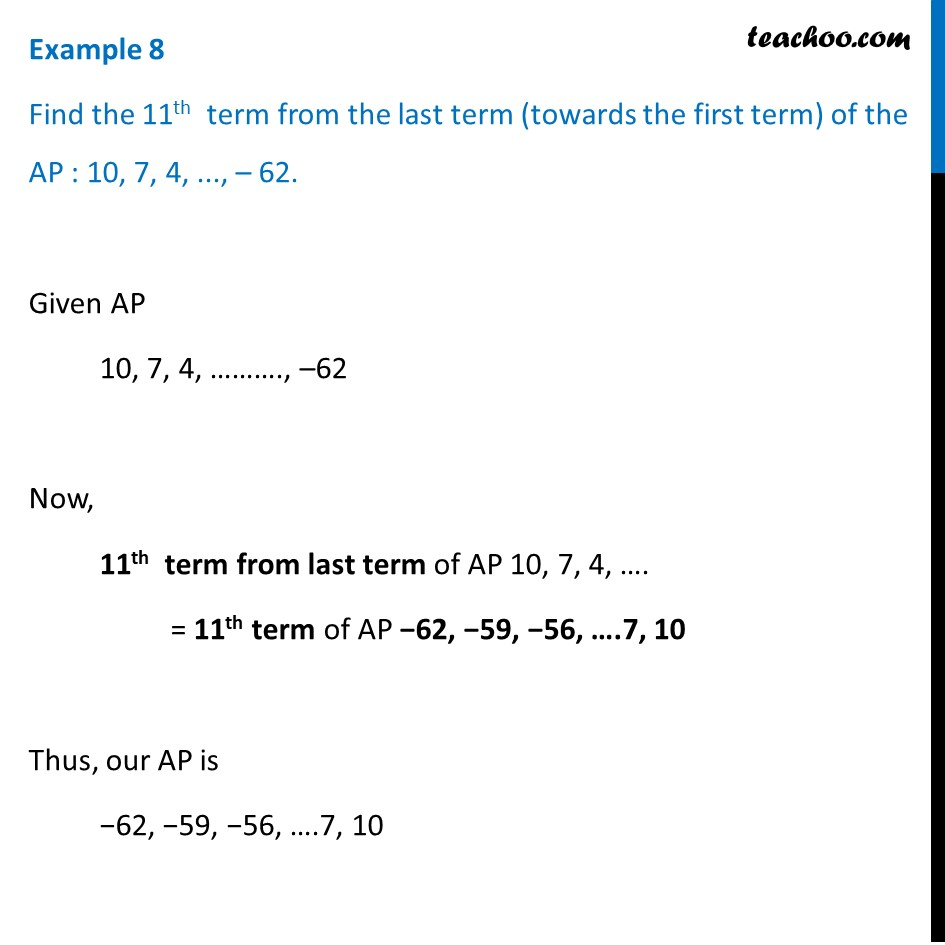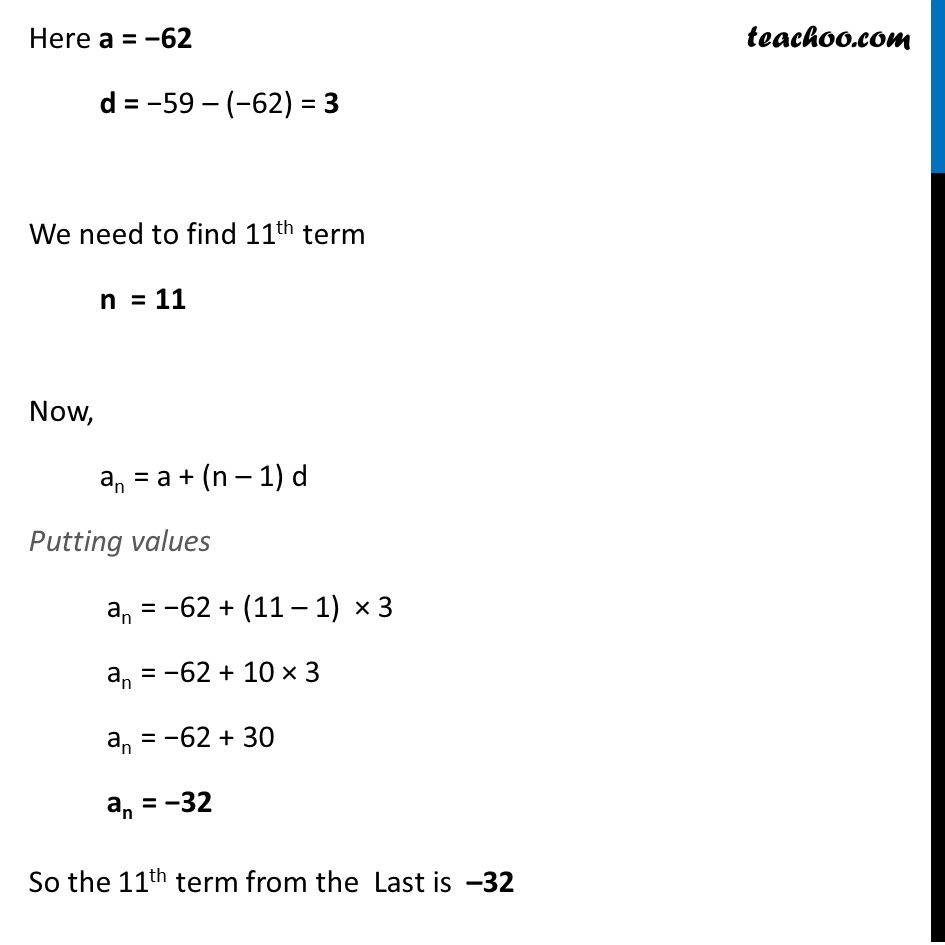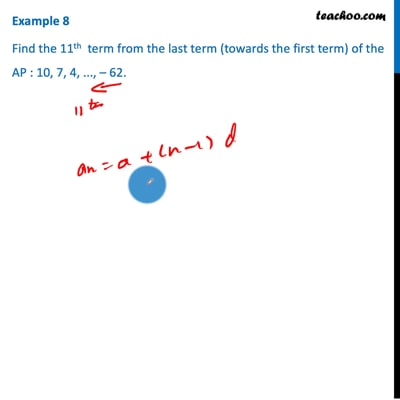Finding nth term from end

Chapter 5 Class 10 Arithmetic Progressions (Term 2)
Concept wiseThis video is only available for Teachoo black users

### Transcript

Example 8 Find the 11th term from the last term (towards the first term) of the AP : 10, 7, 4, ..., – 62. Given AP 10, 7, 4, ………., –62 Now, 11th term from last term of AP 10, 7, 4, …. = 11th term of AP −62, −59, −56, ….7, 10 Thus, our AP is −62, −59, −56, ….7, 10 Here a = −62 d = −59 – (−62) = 3 We need to find 11th term n = 11 Now, an = a + (n – 1) d Putting values an = −62 + (11 – 1) × 3 an = −62 + 10 × 3 an = −62 + 30 an = −32 So the 11th term from the Last is –32